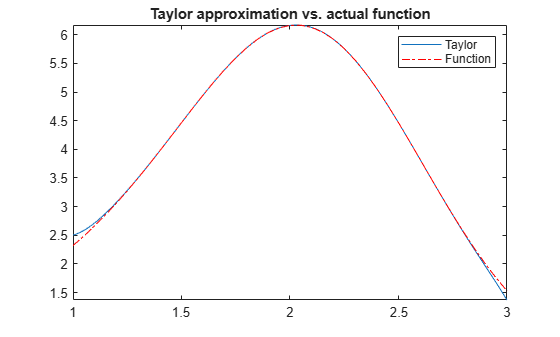## Taylor Series

The statements

```syms x f = 1/(5 + 4*cos(x)); T = taylor(f, 'Order', 8)```

return

```T = (49*x^6)/131220 + (5*x^4)/1458 + (2*x^2)/81 + 1/9```

which is all the terms up to, but not including, order eight in the Taylor series for f(x):

`$\sum _{n=0}^{\infty }{\left(x-a\right)}^{n}\frac{{f}^{\left(n\right)}\left(a\right)}{n!}.$`

Technically, `T` is a Maclaurin series, since its expansion point is `a = 0`.

These commands

```syms x g = exp(x*sin(x)); t = taylor(g, 'ExpansionPoint', 2, 'Order', 12);```

generate the first 12 nonzero terms of the Taylor series for `g` about `x = 2`.

`t` is a large expression; enter

`size(char(t))`
```ans = 1 99791```

to find that `t` has about 100,000 characters in its printed form. In order to proceed with using `t`, first simplify its presentation:

```t = simplify(t); size(char(t))```
```ans = 1 6988```

Next, plot these functions together to see how well this Taylor approximation compares to the actual function `g`:

```xd = 1:0.05:3; yd = subs(g,x,xd); fplot(t, [1, 3]) hold on plot(xd, yd, 'r-.') title('Taylor approximation vs. actual function') legend('Taylor','Function')```Special thanks is given to Professor Gunnar Bäckstrøm of UMEA in Sweden for this example.